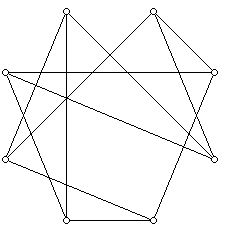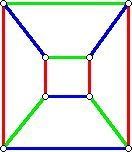N-Cubes Tidbit(11/22/03)

prepared by:

Joseph Malkevitch
Mathematics Department
York College (CUNY)
Jamaica, New York 11451

Email: malkevitch@york.cuny.edu (for additions, suggestions, and corrections)

It is difficult for many people to think about objects in higher dimensional spaces. One way to help understand higher dimensional space is to study concrete examples of objects that exist in such spaces. This note provides some basic information about n-cubes. N-cubes have proved to be a popular architecture for parallel processing computers because of their many nice symmetry properties, and the fact that the distance between any pair of vertices in an n-cube is relatively small considering the large number of vertices that the cube has.

Cubes can be approached metrically or combinatorially. The combinatorial approach defines a n-cube as being constructed from two copies of an (n-1)-cube by joining corresponding vertices by edges. It is traditional to denote the n-cube by Qn.

Starting with a 0-cube, which consists of a single vertex and no edges, the diagram below shows how to construct a 1-cube, then 2-cube from two 1-cubes, and a 3-cube from two 2-cubes.In each case, to get the next larger cube I have drawn two copies of the current cube, and connect the corresponding vertices of the two copies with edges. The construction used can be exploited to develop some "statistical facts" about the n-cubes.

First of all, the number of vertices of an n-cube is going to be twice the number of vertices of an (n-1)-cube.

Thus, we have 1 vertex for a 0-cube, 2 vertices for 1-cube, 4-vertices for a 2-cube and in general, 2n vertices for an n-cube. Furthermore, the vertices of an n-cube are all n-valent. This means there are n edges at each vertex of an n-cube. How many edges does an n-cube have? The number of edges added to the two n-cubes to get an (n+1)-cube is equal to the number of vertices in the n-cube. We can write down a difference equation to express the situation:

E(Qn) = 2E(Qn-1) + V(Qn-1)

This difference equation can be solved by finding a general solution of the difference equation E(Qn) = 2E(Qn-1) and a particular solution of the equation
E(Qn) = 2E(Qn-1) + V(Qn-1). The general solution of the first difference equation is A(2n). A particular solution to the second difference equation is (1/2)(n)(2n).

When the initial condition is applied to determine the single constant in the general solution added to the particular solution we obtain the solution:

E(Qn) = n(2(n-1))

For example, 3(22) = 12 when n is 3, which gives the correct number of edges for the 3-cube as 12.

There are many different ways to represent the graph of a 3-cube:

The diagrams above are quite symmetrical but one can also draw diagrams of the 3-cube that are so unsymmetrical that they make it quite hard to recognize that it is a 3-cube that one is looking at:Note that the 3-cube has a planar graph. Starting with the 4-cube onward, the graphs of the n-cubes are non-planar. There are a variety of open questions concerning the number of edge crossings that can occur for the graph of a cube when it is drawn in the plane.

Among the problems about cubes that remain of research interest is an analysis of the different types of spanning trees that can be drawn on an n-cube. For example, it is not difficult to show that there are 4 different valence vectors that can occur for trees on a 3-cube, but of these only three of the potential types actually exist. The cube below for example shows a spanning tree with two 3-valent vertices, two 2-valent vertices, and four 1-valent vertices.Here is a drawing of the edges of a 3-cube written as the union of three different matchings, drawn in different colors:One can formulate a variety of different questions about matchings of n-cubes which are of research interest. Note that the n-cubes are bipartite graphs. All the circuit lengths are even so that the number of colors needed to color the vertices of an n-cube is only 2.

Below is one way of drawing a 4-dimensional cube:A 5-cube is shown below:One can assign "coordinates" to the vertices of an n-cube using as representatives for the vertices the binary strings of length n. Two of these strings are joined by an edge exactly when they differ in one position. It is easy to see that the n-cubes for n at least 2 have Hamiltonian circuits. A Hamiltonian circuit is a simple closed curve that includes all of the vertices. Since the n-cubes can be coordinatized by the binary strings as described above, it is possible to label 2n objects so that the number of digit changes in going from a description of one of these objects to the next, returning to the first object from the last, is always precisely 1! This means that the labels can be placed so that the total number of digit changes in a cyclic sequence will be exactly 2n. Such codes are known as Gray Codes. Such codes are used in a variety of analog to digital conversion problems.

References

West, D., Introduction to Graph Theory, (second edition), Prentice-Hall, Upper Saddle River, 2001.

Wilf, H., Combinatorial Algorithms: An Update, SIAM, Philadelphia, 1989.

Back to list of tidbits